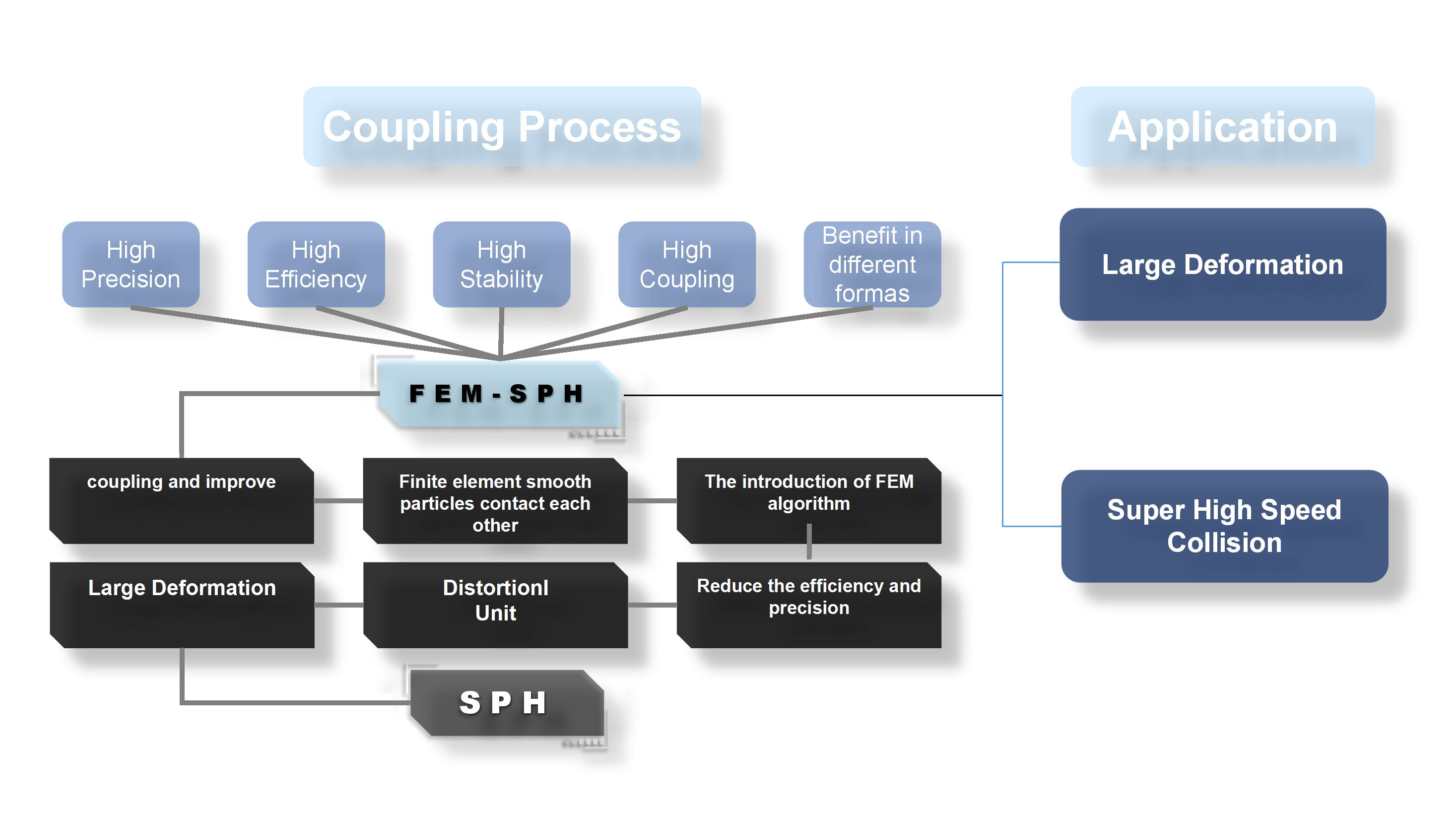Preprint Article Version 1 Preserved in Portico This version is not peer-reviewed

# Numerical Simulation of Hypervelocity Impact FEM-SPH Algorithm Based on Large Deformation of Material

Version 1 : Received: 13 October 2016 / Approved: 14 October 2016 / Online: 14 October 2016 (11:05:45 CEST)

How to cite: Yang, A.; Li, J.; Qu, H.; Pan, Y.; Kang, Y.; Zhang, Y. Numerical Simulation of Hypervelocity Impact FEM-SPH Algorithm Based on Large Deformation of Material. Preprints 2016, 2016100055 (doi: 10.20944/preprints201610.0055.v1). Yang, A.; Li, J.; Qu, H.; Pan, Y.; Kang, Y.; Zhang, Y. Numerical Simulation of Hypervelocity Impact FEM-SPH Algorithm Based on Large Deformation of Material. Preprints 2016, 2016100055 (doi: 10.20944/preprints201610.0055.v1).

## Abstract

In this paper, we first discuss the research status and application progress of the finite element method and the smoothed particle method. By analyzing the advantages of the smoothed particle method and the finite element method, a new coupling algorithm, namely FEM-SPH algorithm, is proposed. By the method of comparison, it shows that finite element method and SPH method in the simulation of large deformation problems each have advantages and disadvantages, the finite element method smoothed particle coupling algorithm is effective to achieve the performance of high computational efficiency and can naturally simulate large deformation problems across. In the process of calculation, the large deformation unit can be freely into an algorithm to facilitate the calculation accuracy and efficiency of three methods of numerical simulation. Through the study found, FEM-SPH algorithm not only overcome the defect of smooth particle tensile instability, but also overcomes the problem of low efficiency of finite element computation. To further test the FEM-SPH algorithm has advantages in the practical engineering, we have carried out the actual test to the example of the super high speed collision, concluded that, since the target of most of the computational domain is always finite element, smoothed particle focused only in contact with the projectile and target of local area, particle number is not much, the whole calculation process just ten minutes, computational efficiency has been greatly improved, at the same time in the simulation of large deformation, the advantage is very obvious .This provides a criterion for the actual project, depending on the specific material deformation mode and choose a more appropriate conversion algorithm.## Keywords

finite element method; smoothed particle method; fem-sph algorithm; large deformation; super high speed collision

## Subject

MATHEMATICS & COMPUTER SCIENCE, Analysis

Views 0